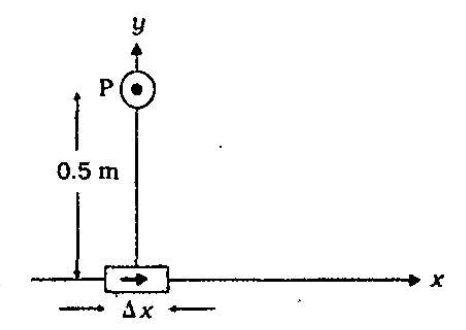Home/Class 12/Physics/

## QuestionPhysicsClass 12

An element $$\Delta l=\Delta x\widehat i$$ is placed at the origin and carries a large current $$I=10A$$. What is the magnetic fleld on the $$y$$-axis at a distance of $$0.5\text{ m}.\Delta x=1\text{ cm}$$$$\mid \mathit{dB}\mid =\frac{\mu _0}{4\pi }\frac{1\mathit{dl}\sin \theta }{r^2}$$
$$\mathit{dt}=\Delta x=10^{-2}m,l=10A,r=0.5m=y{\cdot}\mu _0\text /4\pi =10^{-7}\frac{\mathit{Tm}} A$$
$$\theta =90^{{\circ}}:\sin \theta =1$$
$$\left|\mathit{dB}\right|=\frac{10^{-7}\times 10\times 10^{-2}}{25\times 10^{-2}}=4\times 10^{-8}T$$
The direction of the field is in the $$+z$$  -direction. This is so since. $$\mathit{dl}\times r=\Delta x\widehat i\times y\widehat j=y\Delta x\left(\widehat i\times \widehat j\right)=y\Delta x\widehat k$$
We remind you of the following cyclic property of cross-products. $$\widehat i\times \widehat j=\widehat k;\widehat j\times \widehat k=\widehat i;\widehat k\times \widehat i=\widehat j$$
Note that the field is small in magnitude.